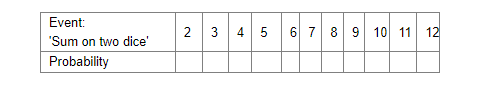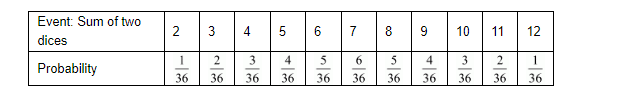# Two dice, one blue and one grey, are thrown at the same time. Complete the following table:

Question:

Two dice, one blue and one grey, are thrown at the same time. Complete the following table:From the above table a student argues that there are 11 possible outcomes $2,3,4,5,6,7,8,9,10,11$ and 12 . Therefore, each of them has a probability $\frac{1}{11}$. Do you agree with this argument?

Solution:

GIVEN: Two dice are thrown

TO FIND: Probability of the following:Let us first write the all possible events that can occur

(1,1), (1,2), (1,3), (1,4), (1,5), (1,6),

(2,1), (2,2), (2,3), (2,4), (2,5), (2,6),

(3,1), (3,2), (3,3), (3,4), (3,5), (3,6),

(4,1), (4,2), (4,3), (4,4), (4,5), (4,6),

(5,1), (5,2), (5,3), (5,4), (5,5), (5,6),

(6,1), (6,2), (6,3), (6,4), (6,5), (6,6),

Hence total number of events is(1) Favorable events i.e. getting the sum of numbers on the dice equal to 2 is

(1, 1),

Hence total number of favorable events i.e. the sum of numbers on the dice equal to 2 is 1

We know that PROBABILITY =Hence probability of getting the sum of numbers on the dice equal to2(2) Favorable events i.e. getting the sum of numbers on the dice equal to 3

are (1,2) and (2,1)

Hence total number of favorable events i.e. the sum of numbers on the dice equal to 3 is 2

We know that PROBABILITY =Hence probability of getting the sum of numbers on the dice equal to 3 =(3) Favorable events i.e. getting the sum of numbers on the dice equal to 4

are (1,3), (2,2) and (3,1)

Hence total number of favorable events i.e. the sum of numbers on the dice equal to 4 is 3

We know that PROBABILITY =Hence probability of getting the sum of numbers on the dice equal to4 is(4) Favorable events i.e. getting the sum of numbers on the dice equal to 5

are (1,4), (2,3), (3,2) and (4,1)

Hence total number of favorable events i.e. getting the sum of numbers on the dice equal to 5 is 4

We know that PROBABILITY =Hence probability of getting the sum of numbers on the dice equal to 5 is(5) Favorable events i.e. getting the total of numbers on the dice equal to 6 are

(1,5), (2,3), (3,3), (4,2) and (5,1)

Hence total number of favorable events i.e. getting the total of numbers on the dice equal to 6 is 5

We know that PROBABILITY =Hence probability of getting the sum of numbers on the dice equal to 6 is(6) Favorable events i.e. getting the sum of both numbers equal to 7 are

(1,6), (2,5), (3,4), (4,3), (5,2) and (6,1)

Hence total number of favorable events i.e. getting the total of numbers on the dice equal to 7 is 6

We know that PROBABILITY =Hence probability of getting the total of numbers on the dice equal to 7 is(7) Favorable events i.e. getting the total of numbers on the dice equal to 8 are

(2,6), (3,5), (4,4), (5,3) and (6,2)

Hence total number of favorable events i.e. getting the total of numbers on the dice equal to 8 is 5

We know that PROBABILITY =Hence probability of getting the total of numbers on the dice equal to 8 is(8) Favorable events i.e. getting the sum of numbers on the dice equal to 9 are

(3,6), (4,5) ,(5,4) and (6,3)

Hence total number of favorable events i.e. getting the sum of numbers on the dice equal to 9 is 4

We know that PROBABILITY =Hence probability of getting the sum of numbers on the dice equal to9 is(9) Favorable events i.e. getting the sum of numbers on the dice equal to 10 are

(4,6), (5,5) and (6,4)

Hence total number of favorable events i.e. the sum of numbers on the dice equal to 10 is 3

We know that PROBABILITY =Hence probability of getting the sum of numbers on the dice equal to10=(10) Favorable events i.e. getting the sum of numbers on the dice equal to 11 are (5, 6) and (6,5),

Hence total number of favorable events i.e. the sum of numbers on the dice equal to 11 is 2

We know that PROBABILITY =Hence probability of getting the sum of numbers on the dice equal to11 is(11) Favorable events i.e. getting the sum of numbers on the dice equal to 12 is

(6, 6)

Hence total number of favorable events i.e. the sum of numbers on the dice equal to 12 is 1

We know that PROBABILITY =Hence probability of getting the sum of numbers on the dice equal to 12The complete table is as followsFrom the above table it is clear that each event does not have same probability